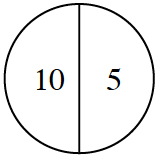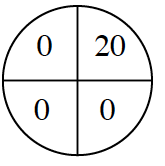### Home > INT2 > Chapter 8 > Lesson 8.3.2 > Problem8-75

8-75.Your teacher has offered your class extra credit. She has created two spinners, shown at right. Your class gets to spin only one of the spinners. The number that the spinner lands on is the number of extra credit points each member of the class will get. Study both spinners carefully.

1. Assuming that each spinner is divided into equal portions, which spinner should the class choose? Justify your answer.

The spinner on the left earns $20$ points only $\frac{1}{4}$ of the time.

The spinner on the right earns $10$ points $\frac{1}{2}$ of the time and $5$ points $\frac{1}{2}$ of the time.

2. What if the spot labeled “$20$” were changed to “$100$”? Now which spinner should the class choose?

Now the spinner on the left earns $100$ points $\frac{1}{4}$ of the time.